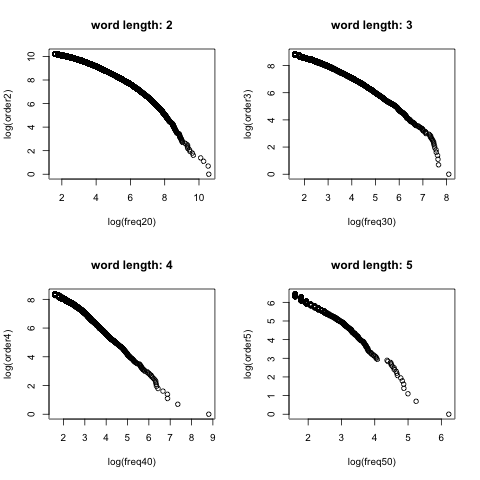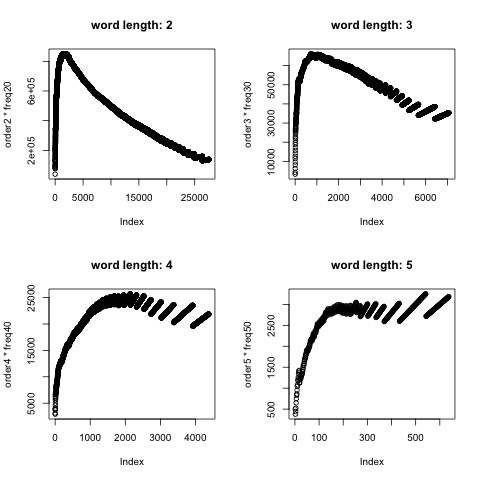2353 字

# 微博是否符合齐普夫定律？## 分析代码

# 读入xml包
library(XML)
# 读取数据并提取文本信息
doc <- xmlTreeParse('NLPIR微博内容语料库.xml',useInternal=TRUE)
rootNode <- xmlRoot(doc)
doc1 <- xpathSApply(rootNode,"//article",xmlValue)
# 去除无关标点与数字
doc2 <- gsub(pattern="http:[a-zA-Z\\/\\.0-9]+","",doc1)
# 中文分词
library(Rwordseg)
doc3 <- segmentCN(doc2)
# 构建语料库 去掉标点与数字与高频词
library(tm)
doc4 <- Corpus(VectorSource(doc3))
doc5 <- tm_map(doc4, removePunctuation)
doc6 <- tm_map(doc5, removeNumbers)
# 高频无意义词在这里可以搞到 https://github.com/yufree/democode/tree/master/data
x <- scan("stopwords.txt", what="")
doc7 <- tm_map(doc6, removeWords, x)
doc8 <- tm_map(doc7, stripWhitespace)
# 构建全范围的词频矩阵
control=list(minDocFreq=5,wordLengths = c(1, Inf),bounds = list(global = c(5,Inf)),weighting = weightTf,encoding = 'UTF-8')
doc.tdm=TermDocumentMatrix(doc8,control)
# 这里截取词频高于5长度为2的词
control2=list(minDocFreq=5,wordLengths = c(2, 2),bounds = list(global = c(5,Inf)),weighting = weightTf,encoding = 'UTF-8')
doc.tdm2=TermDocumentMatrix(doc8,control2)
# 这里截取词频高于5长度为3的词
control3=list(minDocFreq=5,wordLengths = c(3, 3),bounds = list(global = c(5,Inf)),weighting = weightTf,encoding = 'UTF-8')
doc.tdm3=TermDocumentMatrix(doc8,control3)
# 这里截取词频高于5长度为4的词
control4=list(minDocFreq=5,wordLengths = c(4, 4),bounds = list(global = c(5,Inf)),weighting = weightTf,encoding = 'UTF-8')
doc.tdm4=TermDocumentMatrix(doc8,control4)
# 这里截取词频高于5长度为5的词
control5=list(minDocFreq=5,wordLengths = c(5, 5),bounds = list(global = c(5,Inf)),weighting = weightTf,encoding = 'UTF-8')
doc.tdm5=TermDocumentMatrix(doc8,control5)
# 得到词频列表
library(slam)
freq <- rowapply_simple_triplet_matrix(doc.tdm,sum)
freq2 <- rowapply_simple_triplet_matrix(doc.tdm2,sum)
freq3 <- rowapply_simple_triplet_matrix(doc.tdm3,sum)
freq4 <- rowapply_simple_triplet_matrix(doc.tdm4,sum)
freq5 <- rowapply_simple_triplet_matrix(doc.tdm5,sum)
# save(freq,freq2,doc8,file ='constellation.RData')
# 验证齐普夫定律
order <- order(freq[order(freq,decreasing = T)],decreasing = T)
freq0 <- freq[order(freq,decreasing = T)]
order2 <- order(freq2[order(freq2,decreasing = T)],decreasing = T)
freq20 <- freq2[order(freq2,decreasing = T)]
order3 <- order(freq3[order(freq3,decreasing = T)],decreasing = T)
freq30 <- freq3[order(freq3,decreasing = T)]
order4 <- order(freq4[order(freq4,decreasing = T)],decreasing = T)
freq40 <- freq4[order(freq4,decreasing = T)]
order5 <- order(freq5[order(freq5,decreasing = T)],decreasing = T)
freq50 <- freq5[order(freq5,decreasing = T)]
# 结果可视化
# plot(log(order)~log(freq0))
png('logzipfplot.png')
par(mfrow=c(2,2))
plot(log(order2)~log(freq20),main="word length: 2")
plot(log(order3)~log(freq30),main="word length: 3")
plot(log(order4)~log(freq40),main="word length: 4")
plot(log(order5)~log(freq50),main="word length: 5")
dev.off()
png('czipfplot.png')
par(mfrow=c(2,2))
plot(order2*freq20,main="word length: 2")
plot(order3*freq30,main="word length: 3")
plot(order4*freq40,main="word length: 4")
plot(order5*freq50,main="word length: 5")
dev.off()## 现象描述与讨论

zynga 发展中国家      happy      phone
504        189        148        131
webos      china 南京大屠杀      party
130        125        119        108
gmail      world      store      style
106        104         98         98

93         90         89         88
81         79         59         57
weico      weibo      hello      rovio
57         56         53         53
heart 印度尼西亚      icann      green
50         49         49         47
belle      kitty      leave 人民检察院
46         45         45         44

44         44         44         43

42         42         42         42
brian      yahoo 中央气象台      nexon
41         41         40         40
nexus      apple      muddy      still
40         39         39         39
would      ralph
39         38


中国  腐败  城管  一个  北京  微博  问题  政府
38951 37655 28787 24242 15834 14984 12972 12651
社会  今天  美国  国家  工作  公司  经济  已经
11418 11310 11264 11261 10326  9722  8402  8227
现在  时间  表示  事件  香港  发现  世界  发生
8222  7941  7913  7755  7710  7466  7258  7196
进行  知道  人员  安全  生活  目前  新闻  调查
7189  7184  7077  7032  6985  6916  6901  6378
记者  今年  孩子  市场  官员  昨天  事故  企业
6043  6037  6034  5944  5919  5809  5748  5706
看到  图片  朋友  部门  视频  成为  全国  认为
5614  5587  5572  5496  5440  5386  5370  5339
大学  媒体
5317  5219


北京市 越来越 房地产 嫌疑人 公安局 老百姓 互联网
3266   2122   2074   2033   1928   1913   1804

1789   1747   1728   1715   1657   1620   1577

1515   1514   1513   1457   1417   1243   1243

1241   1213   1125   1099   1096   1090   1068

1054   1006    982    952    916    902    897

821    798    796    781    766    764    762

750    741    717    705    688    661    651

642


人心 阻止 一套 原文 随便 展开 男性 下次 无能 征集
421  442  422  443  420  643  421  442  422  441

423  443  420  421  425  442  422  426  643  423

642  441  641  640  420  639  443  440  638  421

425  442  422  426  423  448  444  441  424  420

443  643  440  421  425  641  422  640  442  445


执行官 侦查员 著作权 原材料 总公司    see 男朋友
91     91     91     92     84     63     91

90     92     84     63     91     90     92

84     81     63     89     80     88     83

62     63     91     90     61     81     92

84     89     80     64     62     83     88

63     61     91     90     81     84     71

80     64     87     92     62     89     63

60


## 半成品结论

• 即使进行了高频词的去除，微博环境特别是中文语境也并不符合齐普夫定律
• 中文语料库中展示出的词频与排序山峰式规律可能用来筛选分组中高影响力关键词
• 微博语料库中的中英文混杂现象值得注意
• 欢迎自行探索